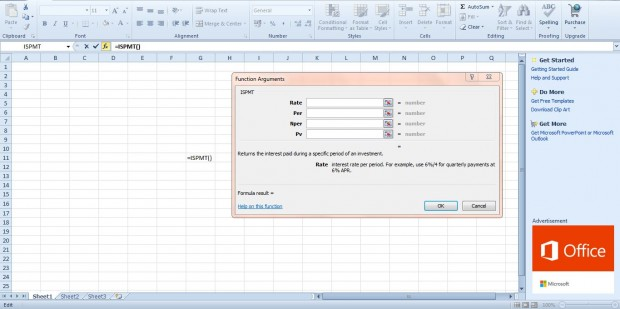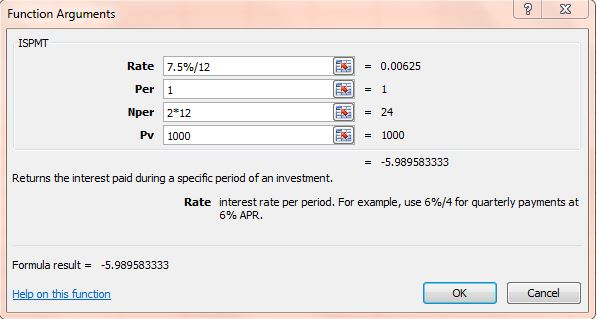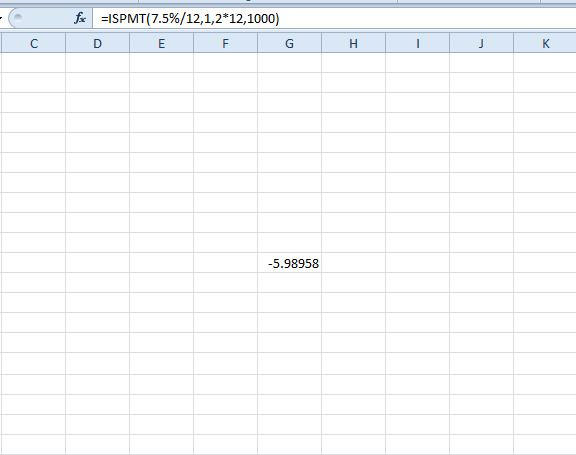# How to use ISPMT function in Excel spreadsheets [Tip]

Excel 2010/13 has a variety of financial functions. Among them is the ISPMT function, which returns the interest paid for a specific period of a loan. The ISPMT sysntax is ISPMT(interest_rate, period, number_payments,PV).

For example, let’s suppose you take out a 1,000 loan with a 7.5% annuel interest rate over a couple of years. With the ISPMT function you can find the interest payment for any of the months. This is how you could find the payment for the first month of the loan period.

You can add this function to a cell without entering data into spreadsheet first. Press the Insert Function (fx) button and select ISPMT to open window below. That includes four fields to enter data in.Enter 7.5%/12 in the Rate field. The interest is divided by 12 for a monthly rate. You could adjust that to 7.5%/4 for quarterly instalments.

Below that input 1 in the Per field, which is the first month of the loan’s period. Enter 2*12 in the Nper field. Enter the loan total of 1000 in the PV field.Then press OK to add the function to the spreadsheet cell. It will return a value of -5.98958. You can find the interest for any period of the loan by entering alternative numbers in the Per field.So with this function you can find out exactly how much of a payment was interest. This video also shows how the ISPMT function is added to Excel spreadsheets.